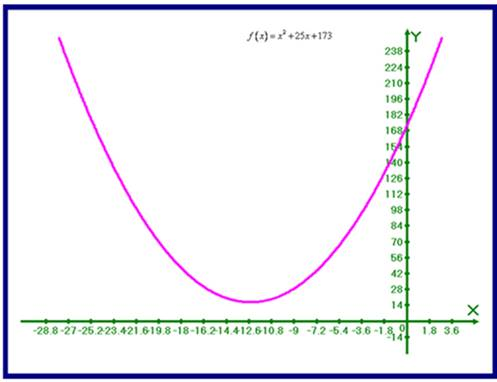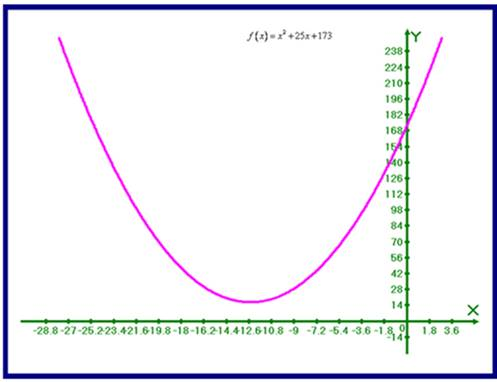# Use a graphical device; we sketch the graph of the function f ( x ) = x 2 + 25 x + 173### Precalculus: Mathematics for Calcu...

6th Edition
Stewart + 5 others
Publisher: Cengage Learning
ISBN: 9780840068071### Precalculus: Mathematics for Calcu...

6th Edition
Stewart + 5 others
Publisher: Cengage Learning
ISBN: 9780840068071

#### Solutions

Chapter 2, Problem 47RE
To determine

## Use a graphical device; we sketch the graph of the function f(x)=x2+25x+173

Expert Solution

Sketch the graph of the function f(x)=x2+25x+173 as shown below:### Explanation of Solution

Given information: consider the function f(x)=x2+25x+173

Calculation:

Use a graphical device; we sketch the graph of the function f(x)=x2+25x+173 as shown below:### Have a homework question?

Subscribe to bartleby learn! Ask subject matter experts 30 homework questions each month. Plus, you’ll have access to millions of step-by-step textbook answers!